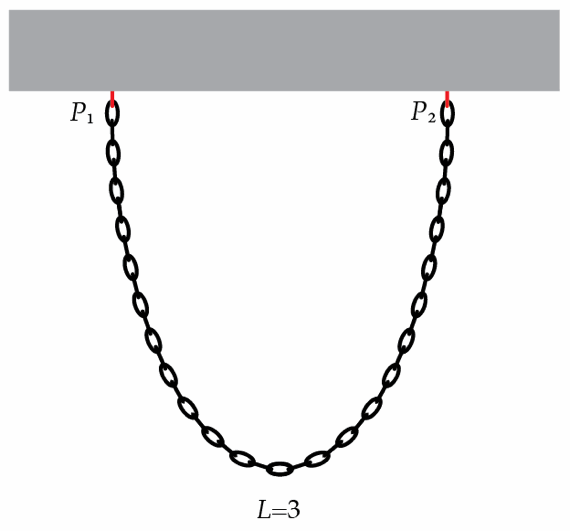# Tension in a hanging chain

A uniform chain of length $L=3~\mbox{m}$ is suspended from two points $P_{1}$ and $P_{2}$ at the same level. Due to the chain's weight, its tension varies from point to point. If the tension at the endpoints of the chain is $n=7$ times greater than the tension at the lowest point, find the distance $d={P_{1}P_{2}}$ in meters between the points of suspension $P_{1}$ and $P_{2}$.×

Problem Loading...

Note Loading...

Set Loading...